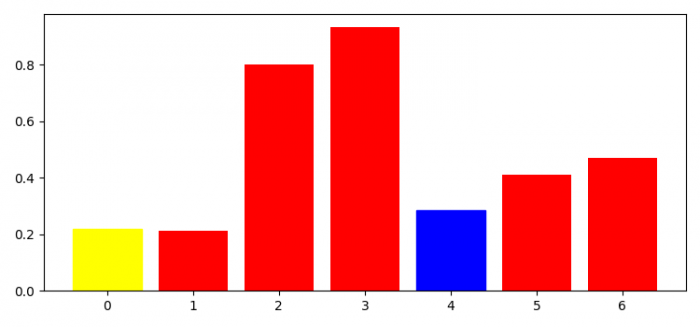# How do I get all the bars in a Matplotlib bar chart?

To get all the bars in a Matplotlib chart, we can use the bar() method and return the bars.−

## Steps

• Set the figure size and adjust the padding between and around the subplots.
• Create a figure and a set of subplots.
• Create x and y data points using subplots() method.
• Make a bar plot and store it in bars variable.
• Set the facecolor of a particular set of bars.
• To display the figure, use show() method.

## Example

import numpy as np
import matplotlib.pyplot as plt

plt.rcParams["figure.figsize"] = [7.50, 3.50]
plt.rcParams["figure.autolayout"] = True

fig, ax = plt.subplots()

x = np.arange(7)
y = np.random.rand(7)

bars = ax.bar(x, y, color='red')
bars.set_color('yellow')
bars.set_color('blue')

plt.show()

## Output